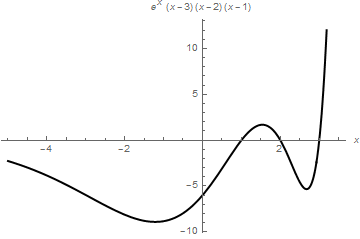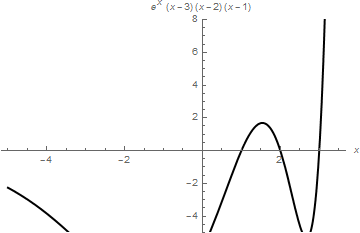# Plotting functions

This chapter demonstrates Mathematica capability to generate graphs. We start with its basic command Plot and expose its ability to add text into figures. To place a text inside a figure, Mathematica has a special command Text[expr, coordinates, offset] that specifies an offset for the block of text relative to the coordinate given. Providing an offset { dx, dy } specifies that the point ( x, y ) should lie at relative coordinates { dx, dy } within the bounding rectangle that encloses the text. We demonstrate it with the following codes:

Plot[Sin[x] - Cos[3*x]/3, {x, 0, 3},
Epilog -> {Text[Style["hello", 25], Scaled[{0.5, 0.5}], #], Red, Point@{.5, .5}},
PlotLabel -> ToString@#] & /@ {{-2.5, 0}, {2.5, 0}, {0, -2}, {0, 2}, {2, 2}, {-3, -2}}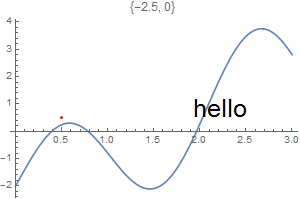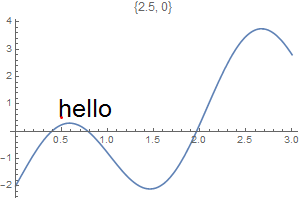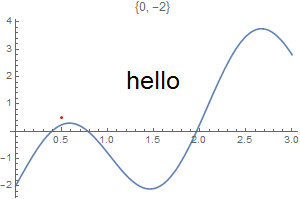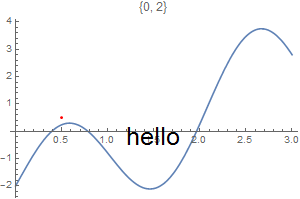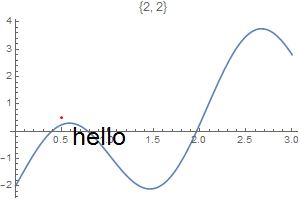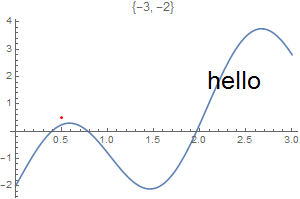However, when we change dimensions of the graph, the text is displayed differently.
Plot[Sin[x] - Cos[3*x]/3, {x, 0, 3},
Epilog -> {Text[Style["hello", 25], Scaled[{0.5, 0.5}], #], Red, Point@{.5, .5}},
PlotLabel -> ToString@#] & /@ {{-2.5, 0}, {2.5, 0}, {0, -2}, {0, 2}, {2, 2}, {-3, -2}}
The following table of graphs can be displayed using GraphicsGrid command. GraphicsGrid by default puts a narrow border around each of the plots in the array it gives. You can change the size of this border by setting the option Spacings -> { h, v} . The parameters h and v give the horizontal and vertical spacings to be used. The Spacings option uses the width and height of characters in the default font to scale the h and v parameters by default, but it is generally more useful in graphics to use Scaled coordinates. Scaled scales widths and heights so that a value of 1 represents the width and height of one element of the grid.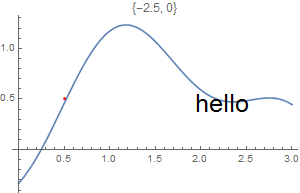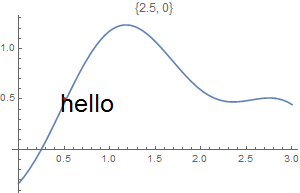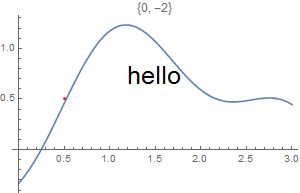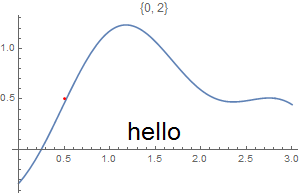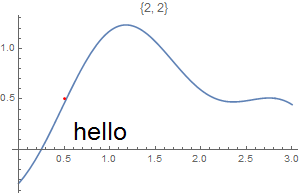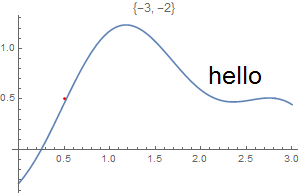How to add text to a graph, see
To add test outside the picture, see
The general reference is

There are someother options.
test1 = Plot[Sin[x] - Cos[3*x]/3, {x, 0, 3}, Epilog -> {Text[Style["World", 25], Scaled[{0.5, 0.5}], #], Red, Point@{.5, .5}}, PlotLabel -> ToString@#] & /@ {{-2.5, 0}, {2.5, 0}, {0, -2}, {0, 2}, {2, 2}, {-3, -2}}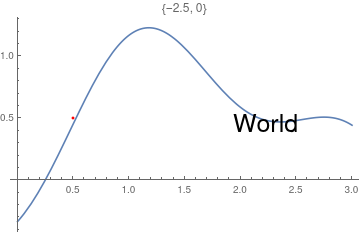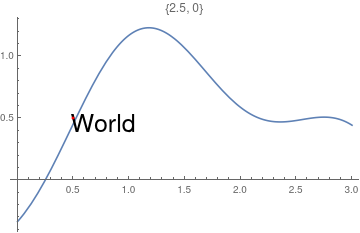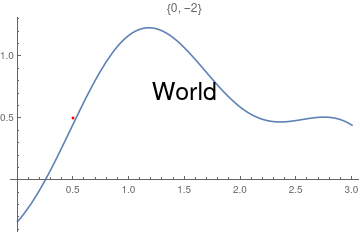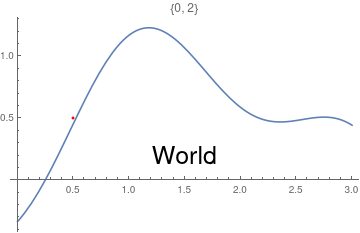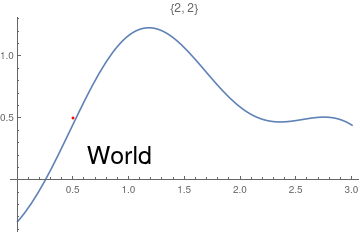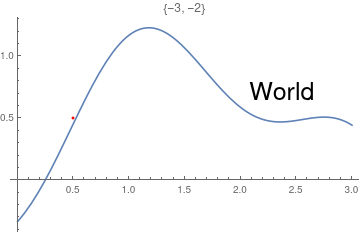test2 = Plot[Sin[x] - Cos[3*x]/3, {x, 0, 3}, Epilog -> {Text[Style["peace", 25], Scaled[{0.5, 0.5}], #], Red, Point@{.5, .5}}, PlotLabel -> ToString@#] & /@ {{-2.5, 0}, {2.5, 0}, {0, -2}, {0, 2}, {2, 2}, {-3, -2}}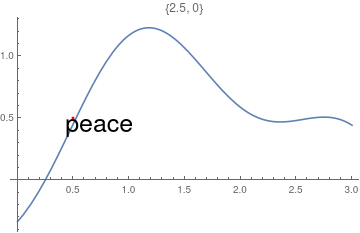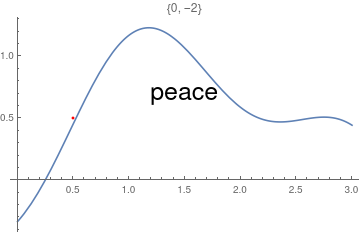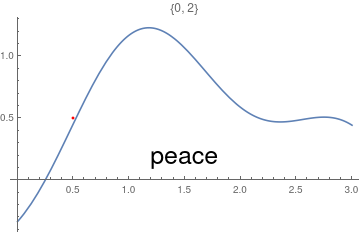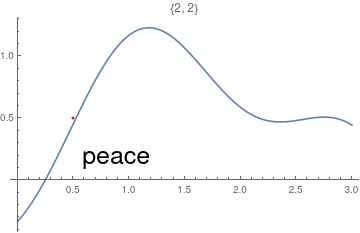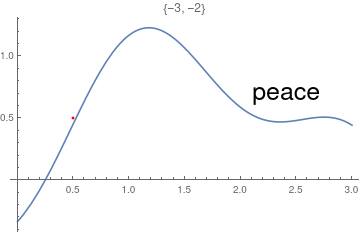TrueQ[test1 == test2]
False

A graphic of a function can be made discrete:

Graphics[{Blue,
Point[Table[{x, Sin[x] - 1/3 Cos[3 x]}, {x, 0, 6, .2}]]}, Axes -> True]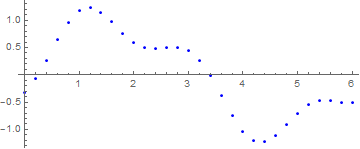We can shift the origin to another point, as the following example shows.
g1[x_] = Sin[x] - 1/3 Cos[3 x]
g1
Out= -1/3
bp = Plot[g1[x], {x, 0, 6}]
Show[bp, AxesOrigin -> {0, -1/3}, AxesLabel -> {"x", "y"}]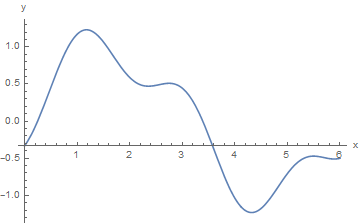When you need to restrict the vertical range, use PlotRange command as the following example shows.

Plot[(x - 1)*(x - 2)*(x - 3)*Exp[x], {x, -5, 3.5}, PlotStyle -> {Black, Thick},
AxesLabel -> {x, (x - 1)*(x - 2)*(x - 3)*Exp[x]}]
Plot[(x - 1)*(x - 2)*(x - 3)*Exp[x], {x, -5, 3.5}, PlotRange -> {-5, 8},
PlotStyle -> {Black, Thick}, AxesLabel -> {x, (x - 1)*(x - 2)*(x - 3)*Exp[x]}]